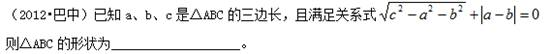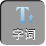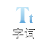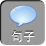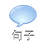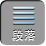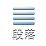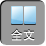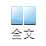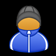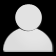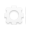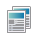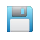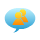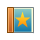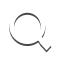-AA+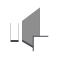_________________________________________________

1、如图，一块草坪的形状为四边形 ABCD，其中∠ B= 90°， AB= 3 cmBC= 4 cmCD= 12 cmAD= 13 cm，求这块草坪的面积．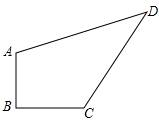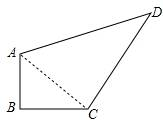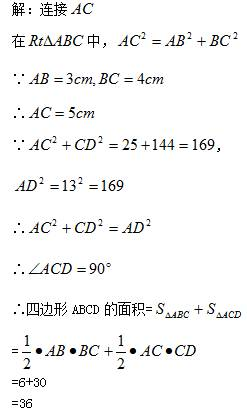2、（ 2004•龙岩）张老师在一次“探究性学习”课中，设计了如下数表：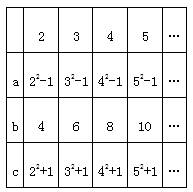1）请你分别观察 abcn之间的关系，并用含自然数 nn1）的代数式表示：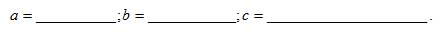2）猜想：以 abc为边的三角形是否为直角三角形并证明你的猜想．

2）分别求出 a 2+ b 2c 2，比较即可．

2）猜想为：以 abc为边的三角形是直角三角形．

a 2+ b 2=（ n 2 -1 2+（ 2 n 2= n 4 -2 n 2+ 1+ 4 n 2= n 4+ 2 n 2+ 1=（ n 2+ 1 2

c 2=（ n 2+ 1 2

∴根据勾股定理的逆定理可知以 abc为边的三角形是直角三角形．

3、已知 abc为△ ABC的三边，且满 a 2 c 2 - b 2 c 2= a 4 - b 4，则△ ABC的形状为____________。

∴（ a 2 - b 2c 2=（ a 2 - b 2）（ a 2+ b 2

∴（ a 2 - b 2）（ a 2+ b 2 - c 2）= 0

a 2 - b 2= 0a 2+ b 2 - c 2= 0

a 2= b 2a 2+ b 2= c 2

∴△ ABC的形状为等腰三角形或直角三角形．